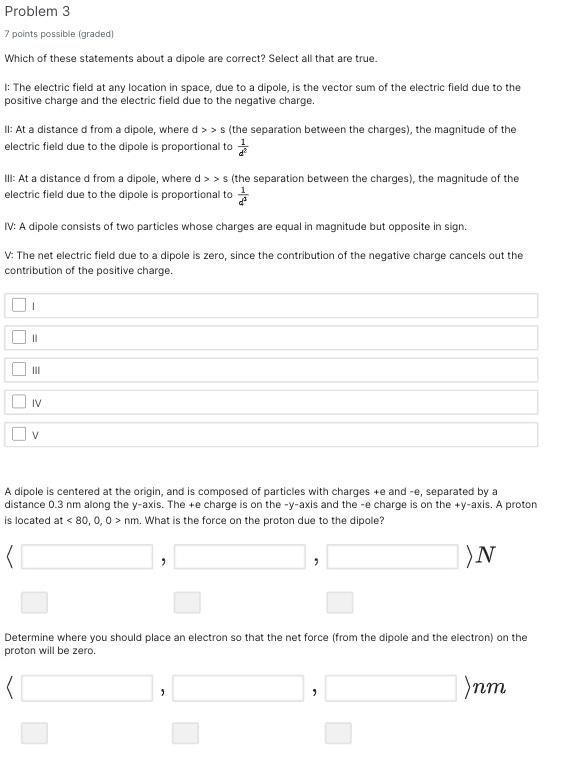# Question Solved1 Answer Problem 3 7 points possible (graded) Which of these statements about a dipole are correct? Select all that are true. l: The electric field at any location in space, due to a dipole, is the vector sum of the electric field due to the positive charge and the electric field due to the negative charge. II: At a distance $$d$$ from a dipole, where $$d>>s$$ Problem 3 7 points possible (graded) Which of these statements about a dipole are correct? Select all that are true. l: The electric field at any location in space, due to a dipole, is the vector sum of the electric field due to the positive charge and the electric field due to the negative charge. II: At a distance d from a dipole, where d>>s (the separation between the charges), the magnitude of the electric field due to the dipole is proportional to d21​ III: At a distance d from a dipole, where d>>s (the separation between the charges), the magnitude of the electric field due to the dipole is proportional to d31​ IV: A dipole consists of two particles whose charges are equal in magnitude but opposite in sign. V. The net electric field due to a dipole is zero, since the contribution of the negative charge cancels out the contribution of the positive charge. II III IV A dipole is centered at the origin, and is composed of particles with charges +e and -e, separated by a distance 0.3 nm along the y-axis. The +e charge is on the -y-axis and the -e charge is on the +y-axis. A proton is located at <80,0,0>nm. What is the force on the proton due to the dipole? \langle , , ⟩N Determine where you should place an electron so that the net force (from the dipole and the electron) on the proton will be zero. \langleTranscribed Image Text: Problem 3 7 points possible (graded) Which of these statements about a dipole are correct? Select all that are true. l: The electric field at any location in space, due to a dipole, is the vector sum of the electric field due to the positive charge and the electric field due to the negative charge. II: At a distance d from a dipole, where d>>s (the separation between the charges), the magnitude of the electric field due to the dipole is proportional to d21​ III: At a distance d from a dipole, where d>>s (the separation between the charges), the magnitude of the electric field due to the dipole is proportional to d31​ IV: A dipole consists of two particles whose charges are equal in magnitude but opposite in sign. V. The net electric field due to a dipole is zero, since the contribution of the negative charge cancels out the contribution of the positive charge. II III IV A dipole is centered at the origin, and is composed of particles with charges +e and -e, separated by a distance 0.3 nm along the y-axis. The +e charge is on the -y-axis and the -e charge is on the +y-axis. A proton is located at <80,0,0>nm. What is the force on the proton due to the dipole? \langle , , ⟩N Determine where you should place an electron so that the net force (from the dipole and the electron) on the proton will be zero. \langle
More
Transcribed Image Text: Problem 3 7 points possible (graded) Which of these statements about a dipole are correct? Select all that are true. l: The electric field at any location in space, due to a dipole, is the vector sum of the electric field due to the positive charge and the electric field due to the negative charge. II: At a distance d from a dipole, where d>>s (the separation between the charges), the magnitude of the electric field due to the dipole is proportional to d21​ III: At a distance d from a dipole, where d>>s (the separation between the charges), the magnitude of the electric field due to the dipole is proportional to d31​ IV: A dipole consists of two particles whose charges are equal in magnitude but opposite in sign. V. The net electric field due to a dipole is zero, since the contribution of the negative charge cancels out the contribution of the positive charge. II III IV A dipole is centered at the origin, and is composed of particles with charges +e and -e, separated by a distance 0.3 nm along the y-axis. The +e charge is on the -y-axis and the -e charge is on the +y-axis. A proton is located at <80,0,0>nm. What is the force on the proton due to the dipole? \langle , , ⟩N Determine where you should place an electron so that the net force (from the dipole and the electron) on the proton will be zero. \langle